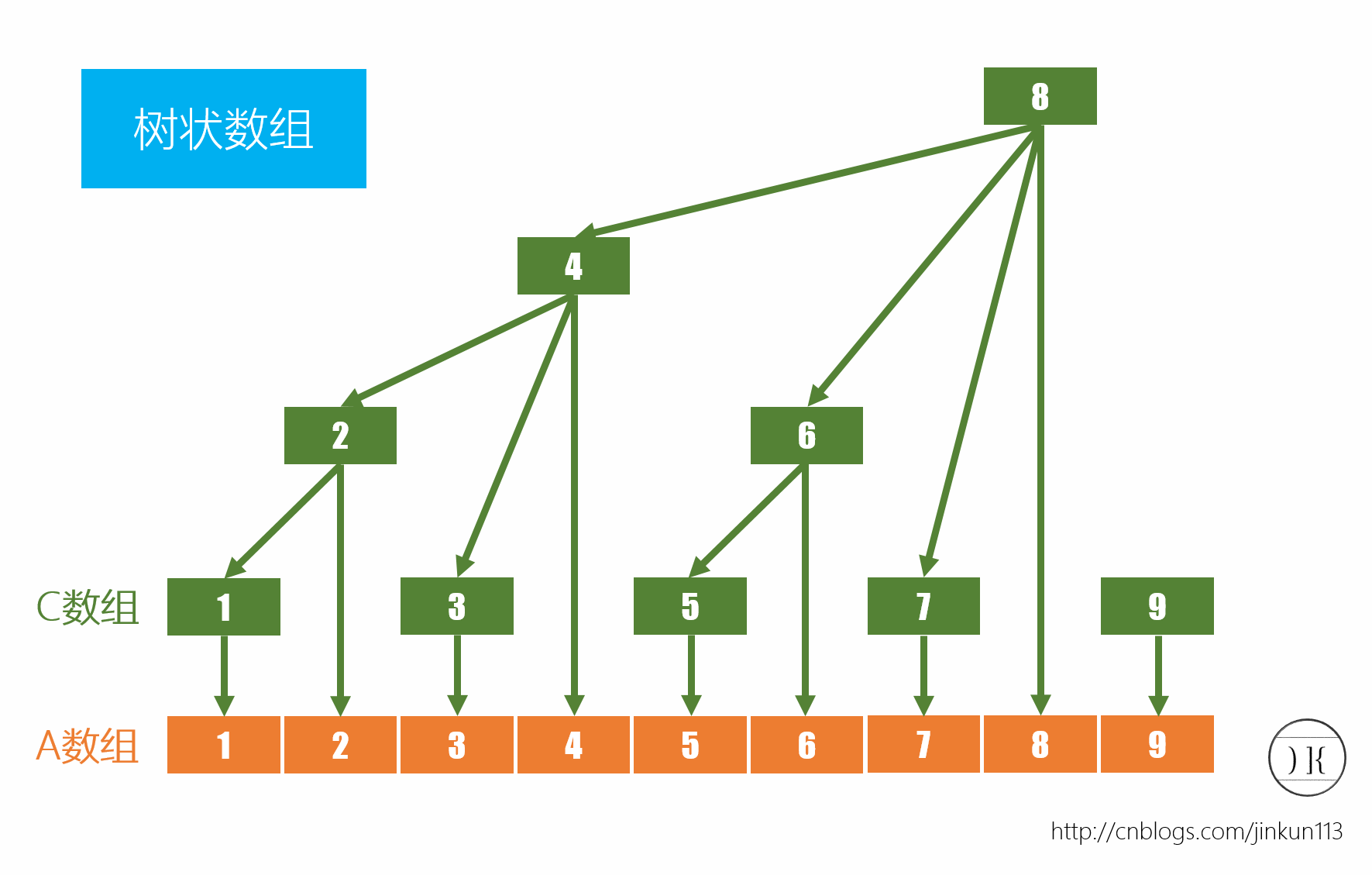# 抱歉，您的浏览器无法访问本站

## 定义

### 特点

• 复杂度：查询和修改的时间复杂度都是$$log(n)$$，空间复杂度则为$$O(n)$$
• 用途：通常用来高效的计算数列的前缀和，区间和，和线段树有点像。
• 可解决的问题：可以解决大部分基于区间上的更新以及求和问题。
• 缺陷：比起线段树来说，在遇到复杂的区间问题还是不能解决。所以说该上线段树还是得上==
• 解题时，应当优先考虑使用树状数组，再考虑使用线段树。

### 图示lowbit： 数字二进制表示的最低1所在位的值。

lowbit返回的值只有一个二进制数字位是1，所以显然它是2的幂。这真的是一个非常巧妙的实现。我是不知道先辈是怎么想出来的，但是又过于理所应当所以不详细解释了。

## 树状数组的变式

### 区间修改+节点查询

• 对差分序列求前缀和可以降低差分序列的阶数，最终得到原序列。
• 原序列[L,R]区间内值+x等价于差分序列中，L处+x，R+1处-x。

• 查询：$$A_i = \sum_{j=1}^i D_j$$
• 修改：$$A_{L\to R}+x \Leftrightarrow D_L+x,D_{R+1}-x$$

### 区间修改+区间查询

$$\sum_{i=1}^nA_i=\sum_{i=1}^n\sum_{j=1}^iD_j$$

$$\sum_{i=1}^nA_i=\sum_{i=1}^n (n+1-i)D_i$$
$$=n\sum_{i=1}^nD_i-\sum_{i=1}^n(i-1)D_i$$

## 练习题

### 洛谷 P3368

UPD: 2021-3-26 已验证板子的正确性；